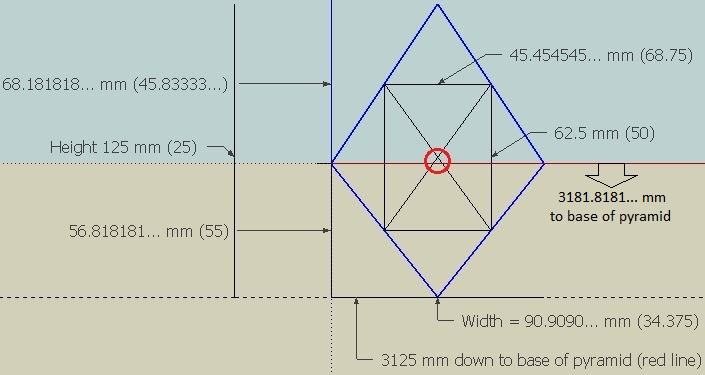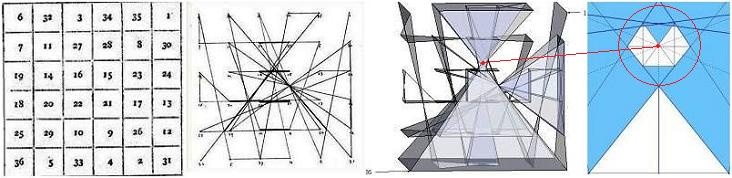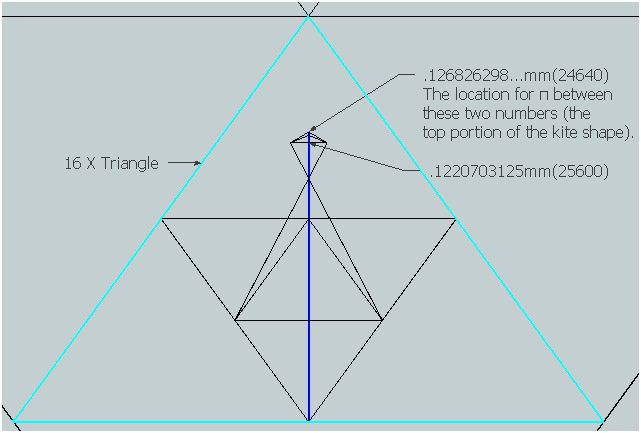Home | Study Links | Poems |

## Pi is by Design

Working within the "Magic Square of the Sun", my goal was to get close to π 3.1415926... by using geometry.
Pi is a fascinating number because it is the mathematical ratio upon which the whole physical universe is designed. This page is a bit lengthy, but it is a record of my progress.The top of the red vertical line at 3125 mm represents the tip of the Pyramid at 3.2
(This concept is best understood after viewing the Sun Square and Square and Circle pages.)

Sitting on top of the pyramid is a 125 mm tall blue diamond shape.
Contained within it π 3.1415926... at 3182.83130505...mm as measured from the base of the pyramid.

##Entering the small blue diamond shape and zeroing in ever closer to π will take up the rest of this page.
(The Babylonians pegged one of the earliest π numbers at 3.125)Several measurements involved in the diamond shape which is as tall as the 125 mm capstone it is sitting on.
Also, 3125 divides the bracketed numbers. For example 3125 / 25= Height 125 mmThe triangle within the red circle enlarged. And, a connection to numbers from the book of Daniel.Before proceeding an interesting feature. The orange lines in the diagram represent the 5x5x5 cube (I used 5000 x 5000 x 5000 mm for this work), red a 6x6x6 cube, and yellow a 7x7x7 cube. Imagine this as a painting with a frame around it. The orange 5x5x5 square represents the inside of the frame, red 6x6x6 the center, and the outside of the frame is represented by the yellow 7x7x7 square. The blue dotted π lines pass through numbers 13 and 18 located on the inside of 5x5x5 frame, the center of the 6x6x6 frame and the outside of the 7x7x7 frame.

Seven represents spiritual perfection, six has to do with man (male and female) the number of imperfection and the human number, five the number for grace, and triple 444 is the world number, and so on. For more on this read Number in Scripture Its Supernatural Design and Spiritual Significance by E. W. Bullinger (1837-1913).

How to calculate the numbers:
From the base of the pyramid to the intersecting blue dotted lines near the location of π is 3181.831325 mm,
add 3125 mm= 6307.831325...2= 39788736.024651255625. Divide this number into 125000000 for answer 3.1415926*337... Correct only to the number 6, but endeavouring to progressively increase its accuracy.

Seeing how the dotted line numbers 13 and 18 intersected close to π, I was reminded of Revelation 13:18 and the
mark of the beast. Verse 18 "Here is wisdom. Let him that hath understanding count the number of the beast:
for it is the number of a man; and his number is Six hundred threescore and six." Literally, the biometric ID number is a “number...that of a man” (Revelation 13:18). "Of a Man Definition: a human being, whether male or female, generically to include "all" human individuals." (Linked Word Project Bible study tool.)

As mentioned elsewhere, accurate measurements and time lines are just one aspect of the Great Pyramid where dates of past and future events written in stone can be measured accurately, and π is a specific point in time.andThese next measurements are from the base of each triangle to the intersecting blue dotted π lines, as they are reduced in size. Shown here are the first two examples: The largest triangle is right side up and has a red post,
the next triangle (reversed) is half the height (not volume) and has a blue post, and so on towards the center.andDiagram A: The triangles lined up so that all are of the same size. Within the fifth triangle (16x) π is now in the upper half of the pyramid, compared to the previous four where it was in the bottom half of each triangle.andDiagram B: As in the previous diagrams the dotted lines do not hit the tops and bottoms of the triangles perfectly. Here the length of each post is based on a set of lines hitting the tops and bottoms of the pyramids perfectly,
as shown in this set of diagrams.This diagram shows the overlay of diagrams A and B. The red lines represent the results from diagram A, and the blue dotted lines represent the results from diagram B. The blue horizontal line is the next area of focus,
and this section is the difference between the results A and B.

To the right and in the 32x triangle is a short green vertical line (circled) of .23873185...mm (13090).The blue horizontal line enlarged and a closer look at the end, the red-circled area.The space .0238731856...mm x " 10 " equals the green line in the previous diagram .23873185...mm (13090).

Pi is located within the .00002516751497...mm space. The method was explained earlier:
From the base of the 3125mm pyramid to the base of the 16x triangle is 3182.70596590...mm (2240625 / 704).
Add .12535939211391...mm (top of the .00002516751497...mm space)= 3182.83132530...mm,
Then divide this number into 125000000 for the answer 3.1415926*334...
The same method for the bottom of the .00002516751497...mm space at .1253342245...mm = 3.14159265*848...
The exact π 3.141592653589... is located between these two numbers.The .00002516751497...mm section enlarged.

.12533914012919...mm = 80.46875%
.12533914130921...mm = 80.46875075757705128436855225383805...%

.12533914012919...mm = 19.53125%
.12533914130921...mm = 19.53125018387792506902149326549467...%The half measurement "0.000286102294921875" is the same number cited in the book De Steenen Spreeken.

80.46875075757705128436855225383805... / 19.53125018387792506902149326549467...= 4.12

Within the unique split # 0.0000000000000293848580... I observed repeating patterns in the strings of numbers
where every section is 12 digits long in 3 out of 4 strings of numbers.97.3554... / 0.0000000000000286077466...= 3403113252010137.6
02.6445... / 0.0000000000000007771114...= 3403113252010137.6

106347289125316800 / 3403113252010137.6= 31.25 x 4= 125

97.35540.../ 4096= Space .023768408... close to π. [4096= 642(Square), (32 Absent), 163(Cube), 84, 46, 212, 1]

97.355401746254475897...% A difference of approximately .05% from π 97.40909103400243723...( π4)

Here is another way to arrange and view the triangles and pi line:Based on previous measurements, the bandwidth in this method is .02754598342...mm (9375 / 340340).
The split (not using the red line) is between .0156093906...(3125 / 200200) and .011936592...mm (3125 / 261800).

The thin red line represents the 13:18 π junction based on the intersecting blue dotted π lines.As mentioned within the 5th triangle (16x), the band width and π are now in the upper half of the pyramid,
compared to the previous four where it was in the bottom half of each triangle.
The thin red line completely misses the 32x triangle and any further triangles from here on.Zeroing further toward the center point π within the triangles using a series of diagrams to reveal a kite shape, and the measurements contained within it. The first drawing shows the previously mentioned blue dotted lines passing through grid points 13 and 18, and in the next drawings joined by other lines that intersect triangle points.Compare to the Tree of Life drawing.Pinpointing π began with the Magic Square of the Sun, and ended at a specific location within the
125 mm tall blue diamond shape set on top of the 3125 mm Pyramid. So, to continue:This unit 0.02029220...mm tall containing π is 154000 times smaller than the 3125 mm tall Pyramid.
Appearing as suspended it is located deep within the diamond shape and it is the focus now.Decreasing the magnification of the 16x triangle to show its location.Several measurements involved in this kite shape!This cube is now the area of focus.Cube side length .0047559862012987012...mm (9375 / 1971200)At .000905902133580... mm ( 9375 / 10348800) the dotted lines lock in the maximum width for this kite shape.Several measurements and ratios that occur within this cube.

Large kite shape:
.0047559862012987012... / .0009059021335807050... = 5.250
.0047559862012987012... / .0018118042671614100... = 2.625
.0038500840677179962...(691.0400390625 / 179487) / .0009059021335807050...(162.59765625 / 179487)= 4.250
.0047559862012987012... .001811804267161410...= .002944181934137291... / .001811804267161410...= 1.625Both side corners of the main kite shape are also kite shaped.The small kite shape has a height divided by width ratio of 2.625
.00007909873134725... (.0455608692560175 / 576) / .00003013285003704861... (0.01735652162134 / 576)= 2.625
.00007909873134725... (.0455608692560175 / 576) / .00001506642501852430... (0.00867826081067 / 576)= 5.250

The red horizontal and vertical π lines is the unique split result between the red and blue numbers:
.00148715739208... and .00326882880921...This diagram based on the previous one has an exaggerated layout so as to better clarify the concepts.
Pi expressed as a .001781671... cube, and like a broken 8 figure, flows/loops around the peripheral.
Or from an artists perspective, a butterfly and its reflection.
.00148715739208... x 2= .00297431478417...
.00475598620129... minus .00297431478417...= .00178167141712...mm

This work describes the point of division of the circling vortex, or spin, of the electromagnetic energies of the north and south poles (visualize an hourglass). Called a Bloch Wall, it is a neutral center gravity wave source, and the gap between opposite magnet faces, the junction of two magnetic poles (north and south). It is a magnetic flow reversal point also known as a diamagnetic vortex point.

The various lightspeed values reflect force of gravity and increasing mass where distance contracts and time is slowed. All speed of light measurements thus far have been influenced by earth gravity.
(The current universal speed of light is rounded off to 186282.397 mps.)
It resides between the small kites’ center and its exterior at 186282.372...mps and 186282.407...mps respectively.

Aproximate speed of light numbers:
I
The small kite shapes' center pinpoints a lightspeed of 186282.3722...mps
299792418.110119...(6295640780.3125 / 21) meters per second
II
299792457.985119...(6295641617.6875 / 21) meters per second= 186282.3970425...mps
III
301411.25 / Phi 1.618...= 186282.3970915...mps
IV
c = 186282 + (1.25 / π) perhaps

A method to calculate π:

0.1268262987012... ( 3125 / 24640 ) (Diagram at the top of this page)
subtract
.00148715739208...
=
0.1253391413092...
+
57.705965909090... ( 1421875 / 24640 )
=
57.831305050400...
+
6250 (3125 x 2)
=
6307.8313050504...
Squared =
39788735.7729738339...
Divide into:
125000000
=
π 3.141592653589...

Square and CircleSplitting A to B linear distances down the middle forever theoretically and adding more and more digits to π was an early geometrical approach. Around 250 BC the Greek mathematician Archimedes began estimating π using circumscribed and inscribed polygons. However, think of pi as 3-dimensional not 2-d as in pie shaped.
We always face a spiral, never a circle.

3125 + 57.831305...= 3182.83130505...mm from base of pyramid is the exact location for π within the diamond.

This information is contained in an unassuming looking Magic Square of the Sun design, reputed to be many thousands of years old. To have the knowhow to design something like this, and make everything fit so perfect is something. Yet it exists, and in conclusion π 3.141592653... the mathematical ratio upon which the whole physical universe was designed, is not just a collection of random numbers caused by a chaotic and ongoing "Big Bang" explosion as is presumed today, but was perfectly and deliberately designed.

Unfortunately, "Sometimes people hold a core belief that is very strong. When they are presented with evidence that works against that belief, the new evidence cannot be accepted. It would create a feeling that is extremely uncomfortable, called cognitive dissonance. And because it is so important to protect the core belief, they will rationalize, ignore, and even deny anything that doesn't fit in with the core belief." - Frantz Fanon"Reality (time) is merely an illusion, albeit a very persistent one."
Albert Einstein